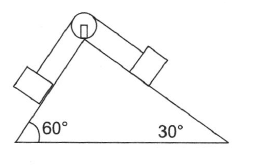Two blocks of equal mass are tied with a light string which passes over a massless pulley as shown in figure. The magnitude of acceleration of centre of mass of both the blocks is (neglect friction every where):

# Two blocks of equal mass are tied with a light string which passes over a massless pulley as shown in figure. The magnitude of acceleration of centre of mass of both the blocks is (neglect friction every where):1. A

$\frac{\sqrt{3}-1}{4\sqrt{2}}\mathrm{g}$

2. B

$\left(\sqrt{3}-1\right)\mathrm{g}$

3. C

$\frac{\mathrm{g}}{2}$

4. D

$\left(\frac{\sqrt{3}-1}{\sqrt{2}}\right)\mathrm{g}$

Register to Get Free Mock Test and Study Material

+91

Verify OTP Code (required)

### Solution:

mg sin

Solving them, we get

Now

= $\frac{\mathrm{a}}{4}\left[\left(1+\sqrt{3}\right)\stackrel{^}{\mathrm{i}}+\left(1-\sqrt{3}\right)\stackrel{^}{\mathrm{j}}\right]$Register to Get Free Mock Test and Study Material

+91

Verify OTP Code (required)